The Kinetic Molecular Theory

The Kinetic Molecular Theory Postulates

The experimental observations about the behavior of gases discussed so far can be explained with a simple theoretical model known as the kinetic molecular theory. This theory is based on the following postulates, or assumptions.

1. Gases are composed of a large number of particles that behave like hard, spherical objects in a state of constant, random motion.
2. These particles move in a straight line until they collide with another particle or the walls of the container.
3. These particles are much smaller than the distance between particles. Most of the volume of a gas is therefore empty space.
4. There is no force of attraction between gas particles or between the particles and the walls of the container.
5. Collisions between gas particles or collisions with the walls of the container are perfectly elastic. None of the energy of a gas particle is lost when it collides with another particle or with the walls of the container.
6. The average kinetic energy of a collection of gas particles depends on the temperature of the gas and nothing else.

The assumptions behind the kinetic molecular theory can be illustrated with the apparatus shown in the figure below, which consists of a glass plate surrounded by walls mounted on top of three vibrating motors. A handful of steel ball bearings are placed on top of the glass plate to represent the gas particles.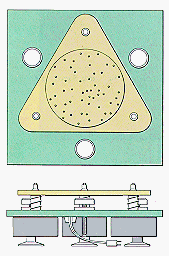When the motors are turned on, the glass plate vibrates, which makes the ball bearings move in a constant, random fashion (postulate 1). Each ball moves in a straight line until it collides with another ball or with the walls of the container (postulate 2). Although collisions are frequent, the average distance between the ball bearings is much larger than the diameter of the balls (postulate 3). There is no force of attraction between the individual ball bearings or between the ball bearings and the walls of the container (postulate 4).

The collisions that occur in this apparatus are very different from those that occur when a rubber ball is dropped on the floor. Collisions between the rubber ball and the floor are inelastic, as shown in the figure below. A portion of the energy of the ball is lost each time it hits the floor, until it eventually rolls to a stop. In this apparatus, the collisions are perfectly elastic. The balls have just as much energy after a collision as before (postulate 5).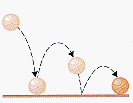Any object in motion has a kinetic energy that is defined as one-half of the product of its mass times its velocity squared.

KE = 1/2 mv2

At any time, some of the ball bearings on this apparatus are moving faster than others, but the system can be described by an average kinetic energy. When we increase the "temperature" of the system by increasing the voltage to the motors, we find that the average kinetic energy of the ball bearings increases (postulate 6).How the Kinetic Molecular Theory Explains the Gas Laws

The kinetic molecular theory can be used to explain each of the experimentally determined gas laws.

The Link Between P and n

The pressure of a gas results from collisions between the gas particles and the walls of the container. Each time a gas particle hits the wall, it exerts a force on the wall. An increase in the number of gas particles in the container increases the frequency of collisions with the walls and therefore the pressure of the gas.

Amontons' Law (P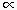T)

The last postulate of the kinetic molecular theory states that the average kinetic energy of a gas particle depends only on the temperature of the gas. Thus, the average kinetic energy of the gas particles increases as the gas becomes warmer. Because the mass of these particles is constant, their kinetic energy can only increase if the average velocity of the particles increases. The faster these particles are moving when they hit the wall, the greater the force they exert on the wall. Since the force per collision becomes larger as the temperature increases, the pressure of the gas must increase as well.

Boyle's Law (P = 1/v)

Gases can be compressed because most of the volume of a gas is empty space. If we compress a gas without changing its temperature, the average kinetic energy of the gas particles stays the same. There is no change in the speed with which the particles move, but the container is smaller. Thus, the particles travel from one end of the container to the other in a shorter period of time. This means that they hit the walls more often. Any increase in the frequency of collisions with the walls must lead to an increase in the pressure of the gas. Thus, the pressure of a gas becomes larger as the volume of the gas becomes smaller.

Charles' Law (VT)

The average kinetic energy of the particles in a gas is proportional to the temperature of the gas. Because the mass of these particles is constant, the particles must move faster as the gas becomes warmer. If they move faster, the particles will exert a greater force on the container each time they hit the walls, which leads to an increase in the pressure of the gas. If the walls of the container are flexible, it will expand until the pressure of the gas once more balances the pressure of the atmosphere. The volume of the gas therefore becomes larger as the temperature of the gas increases.

Avogadro's Hypothesis (VN)

As the number of gas particles increases, the frequency of collisions with the walls of the container must increase. This, in turn, leads to an increase in the pressure of the gas. Flexible containers, such as a balloon, will expand until the pressure of the gas inside the balloon once again balances the pressure of the gas outside. Thus, the volume of the gas is proportional to the number of gas particles.

Dalton's Law of Partial Pressures (Pt = P1 + P2 + P3 + ...)

Imagine what would happen if six ball bearings of a different size were added to the molecular dynamics simulator. The total pressure would increase because there would be more collisions with the walls of the container. But the pressure due to the collisions between the original ball bearings and the walls of the container would remain the same. There is so much empty space in the container that each type of ball bearing hits the walls of the container as often in the mixture as it did when there was only one kind of ball bearing on the glass plate. The total number of collisions with the wall in this mixture is therefore equal to the sum of the collisions that would occur when each size of ball bearing is present by itself. In other words, the total pressure of a mixture of gases is equal to the sum of the partial pressures of the individual gases.Graham's Laws of Diffusion and Effusion

A few of the physical properties of gases depend on the identity of the gas. One of these physical properties can be seen when the movement of gases is studied.

In 1829 Thomas Graham used an apparatus similar to the one shown in the figure below to study the diffusion of gasesthe rate at which two gases mix. This apparatus consists of a glass tube sealed at one end with plaster that has holes large enough to allow a gas to enter or leave the tube. When the tube is filled with H2 gas, the level of water in the tube slowly rises because the H2 molecules inside the tube escape through the holes in the plaster more rapidly than the molecules in air can enter the tube. By studying the rate at which the water level in this apparatus changed, Graham was able to obtain data on the rate at which different gases mixed with air.Graham found that the rates at which gases diffuse is inversely proportional to the square root of their densities.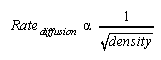This relationship eventually became known as Graham's law of diffusion.

To understand the importance of this discovery we have to remember that equal volumes of different gases contain the same number of particles. As a result, the number of moles of gas per liter at a given temperature and pressure is constant, which means that the density of a gas is directly proportional to its molecular weight. Graham's law of diffusion can therefore also be written as follows.Similar results were obtained when Graham studied the rate of effusion of a gas, which is the rate at which the gas escapes through a pinhole into a vacuum. The rate of effusion of a gas is also inversely proportional to the square root of either the density or the molecular weight of the gas.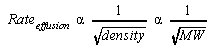Graham's law of effusion can be demonstrated with the apparatus in the figure below. A thick-walled filter flask is evacuated with a vacuum pump. A syringe is filled with 25 mL of gas and the time required for the gas to escape through the syringe needle into the evacuated filter flask is measured with a stop watch.As we can see when data obtained in this experiment are graphed in the figure below, the time required for 25-mL samples of different gases to escape into a vacuum is proportional to the square root of the molecular weight of the gas. The rate at which the gases effuse is therefore inversely proportional to the square root of the molecular weight. Graham's observations about the rate at which gases diffuse (mix) or effuse (escape through a pinhole) suggest that relatively light gas particles such as H2 molecules or He atoms move faster than relatively heavy gas particles such as CO2 or SO2 molecules.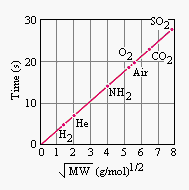The Kinetic Molecular Theory and Graham's Laws

The kinetic molecular theory can be used to explain the results Graham obtained when he studied the diffusion and effusion of gases. The key to this explanation is the last postulate of the kinetic theory, which assumes that the temperature of a system is proportional to the average kinetic energy of its particles and nothing else. In other words, the temperature of a system increases if and only if there is an increase in the average kinetic energy of its particles.

Two gases, such as H2 and O2, at the same temperature, therefore must have the same average kinetic energy. This can be represented by the following equation.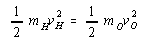This equation can be simplified by multiplying both sides by two.It can then be rearranged to give the following.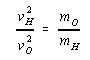Taking the square root of both sides of this equation gives a relationship between the ratio of the velocities at which the two gases move and the square root of the ratio of their molecular weights.This equation is a modified form of Graham's law. It suggests that the velocity (or rate) at which gas molecules move is inversely proportional to the square root of their molecular weights.

 Practice Problem 14:Calculate the average velocity of an H2 molecule at 0° C if the average velocity of an O2   molecule at this temperature is 500 m/s. Click here to check your answer to Practice Problem 14. Click here to see a solution to Practice Problem 14.